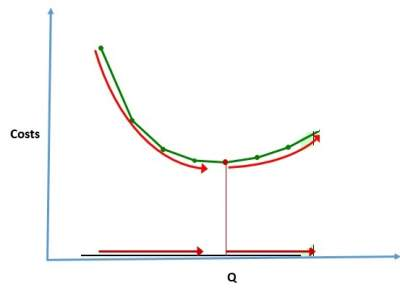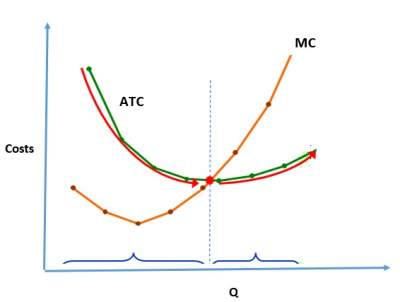# Fixed and Variable Costs

## Introduction

• Fixed costs (FC) will remain the same regardless of the quantity of output produced such as rent.
• On the other hand, variable costs (VC) vary with the quantity produced such as salaries.
• Total cost (TC) = FC + VC

### Average Fixed Cost, Average Variable Cost, and Average Total Cost

• Average Fixed Cost (AFC) = FC / Q
• Average Variable Cost (AVC) = AVC / Q
• Average Total Cost (ATC) = TC / Q = AFC + AVC

### Exercise

Exercise on Calculating costs

 As Q increases: in the beginning, AFC decreases which pulls down ATC. Then as Q keeps increasing, AVC rises as well as ATC. Hence, to be efficient, we need to find Q that minimizes ATC.When MC < ATC, ATC decreases. But when MC > ATC, ATC rises. The MC curve crosses the ATC curve at the ATC curve's minimum.